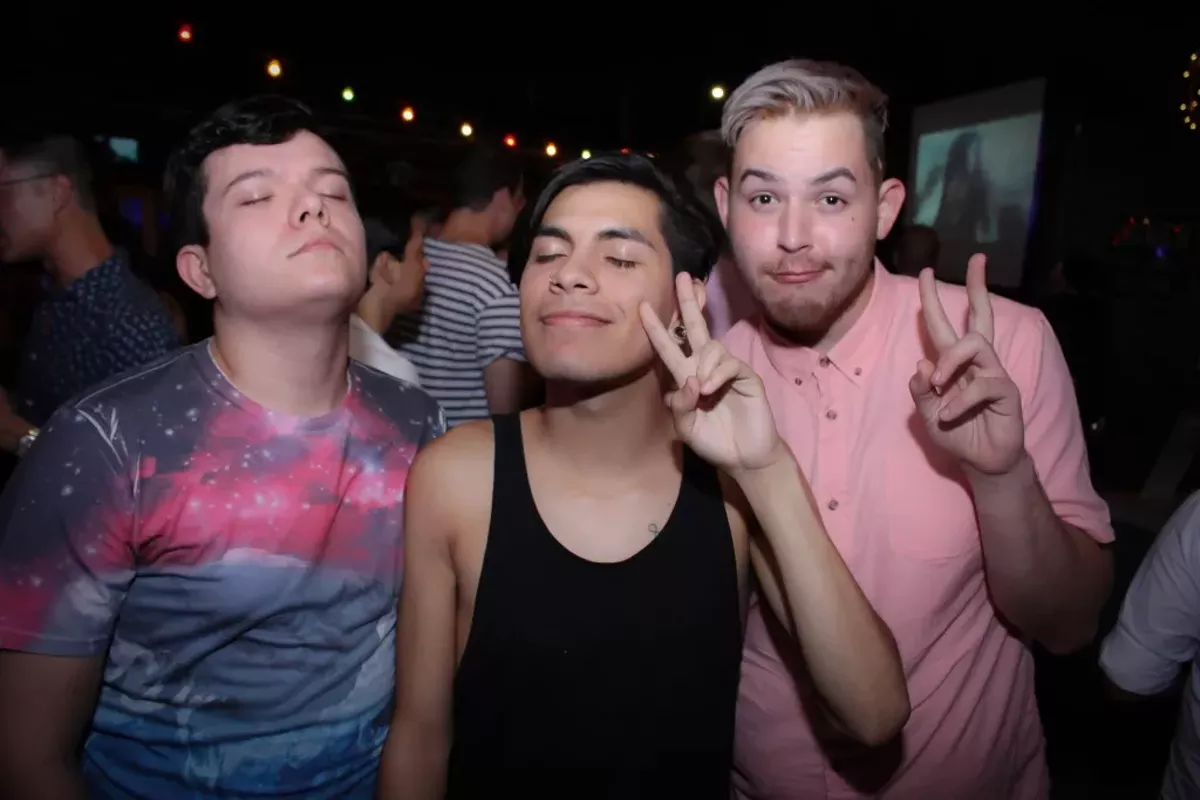# 52 Glamorous Moments from Beyonce's Birthday Bash at Brass Monkey

Tweet
Submit to Reddit
Email
Photos by Julian P. Ledezma
OF 52
PREV NEXTJulian P. Ledezma
Julian P. Ledezma
Julian P. Ledezma
Julian P. Ledezma
Julian P. Ledezma
Julian P. Ledezma
Julian P. Ledezma
Julian P. Ledezma
Julian P. Ledezma
Julian P. Ledezma
Julian P. Ledezma
Julian P. Ledezma
Julian P. Ledezma
Julian P. Ledezma
Julian P. Ledezma
Julian P. Ledezma
Julian P. Ledezma
Julian P. Ledezma
Julian P. Ledezma
Julian P. Ledezma
Julian P. Ledezma
Julian P. Ledezma
Julian P. Ledezma
Julian P. Ledezma
Julian P. Ledezma
Julian P. Ledezma
Julian P. Ledezma
Julian P. Ledezma
Julian P. Ledezma
Julian P. Ledezma
Julian P. Ledezma
Julian P. Ledezma
Julian P. Ledezma
Julian P. Ledezma
Julian P. Ledezma
Julian P. Ledezma
Julian P. Ledezma
Julian P. Ledezma
Julian P. Ledezma
Julian P. Ledezma
Julian P. Ledezma
Julian P. Ledezma
Julian P. Ledezma
Julian P. Ledezma
Julian P. Ledezma
Julian P. Ledezma
Julian P. Ledezma
Julian P. Ledezma
Julian P. Ledezma
Julian P. Ledezma